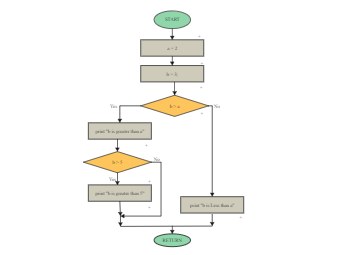Template Community / The Algorithm Flowchart

The Algorithm Flowchart

Share
Duplicate0
0
Report
Publish time：07-16-2021
Algorithms are used to find the most significant number among the two but following some basic steps. Assuming the numbers are input one after the other, create a variable called largest to store the most significant number. When the first number is input, set the variable' most significant' equal to that number. As the following two numbers are input, check to see if the number that was input is greater than the variable stored in 'largest.' If the number that was input is more significant, then-largest is set equal to the number that was input. After the three numbers have been input, the number stored in 'largest' will be the largest. As shown in the below algorithm, if the entered value of b is more excellent than a, then the System will print "b is greater than a," else it will print "b is less than a."
CreatorCharlottePost
Recommended Templates